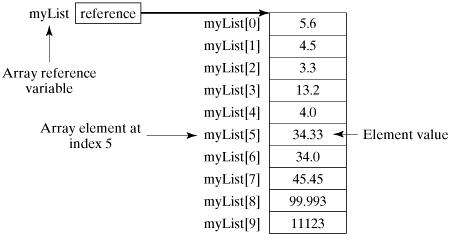# Powershell - Array

PowerShell provides a data structure, the array, which stores a fixed-size sequential collection of elements of the any type. An array is used to store a collection of data, but it is often more useful to think of an array as a collection of variables or objects.

Instead of declaring individual variables, such as number0, number1, ..., and number99, you declare one array variable such as numbers and use numbers, numbers, and ..., numbers to represent individual variables.

This tutorial introduces how to declare array variables, create arrays, and process arrays using indexed variables.

## Declaring Array Variables

To use an array in a program, you must declare a variable to reference the array, and you can specify the type of array the variable can reference. Here is the syntax for declaring an array variable −

### Syntax

```\$A = 1, 2, 3, 4
or
\$A = 1..4
```

Note − By default type of objects of array is System.Object. GetType() method returns the type of the array. Type can be passed.

### Example

The following code snippets are examples of this syntax −

```[int32[]]\$intA = 1500,2230,3350,4000

\$A = 1, 2, 3, 4
\$A.getType()
```

This will produce the following result −

## Output

```IsPublic    IsSerial    Name                        BaseType
--------    --------    ----                        --------
True        True        Object[]                    System.Array
```

The array elements are accessed through the index. Array indices are 0-based; that is, they start from 0 to arrayRefVar.length-1.

### Example

Following statement declares an array variable, myList, creates an array of 10 elements of double type and assigns its reference to myList −

```\$myList = 5.6, 4.5, 3.3, 13.2, 4.0, 34.33, 34.0, 45.45, 99.993, 11123
```

Following picture represents array myList. Here, myList holds ten double values and the indices are from 0 to 9.## Processing Arrays

When processing array elements, we often use either for loop or foreach loop because all of the elements in an array are of the same type and the size of the array is known.

### Example

Here is a complete example showing how to create, initialize, and process arrays −

```\$myList = 5.6, 4.5, 3.3, 13.2, 4.0, 34.33, 34.0, 45.45, 99.993, 11123

write-host("Print all the array elements")
\$myList

write-host("Get the length of array")
\$myList.Length

write-host("Get Second element of array")
\$myList

write-host("Get partial array")
\$subList = \$myList[1..3]

write-host("print subList")
\$subList

write-host("using for loop")
for (\$i = 0; \$i -le (\$myList.length - 1); \$i += 1) {
\$myList[\$i]
}

write-host("using forEach Loop")
foreach (\$element in \$myList) {
\$element
}

write-host("using while Loop")
\$i = 0
while(\$i -lt 4) {
\$myList[\$i];
\$i++
}

write-host("Assign values")
\$myList = 10
\$myList
```

This will produce the following result −

### Output

```Print all the array elements
5.6
4.5
3.3
13.2
4
34.33
34
45.45
99.993
11123
Get the length of array
10
Get Second element of array
4.5
Get partial array
print subList
4.5
3.3
13.2
using for loop
5.6
4.5
3.3
13.2
4
34.33
34
45.45
99.993
11123
using forEach Loop
5.6
4.5
3.3
13.2
4
34.33
34
45.45
99.993
11123
using while Loop
5.6
4.5
3.3
13.2
Assign values
5.6
10
3.3
13.2
4
34.33
34
45.45
99.993
11123
```

## The Arrays Methods Examples

Here is a complete example showing operations on arrays using its methods

```\$myList = @(0..4)

write-host("Print array")
\$myList

\$myList = @(0..4)

write-host("Assign values")
\$myList  = 10
\$myList
```

This will produce the following result −

### Output

```Clear array
Print array
0
1
2
3
4
Assign values
0
10
2
3
4
```